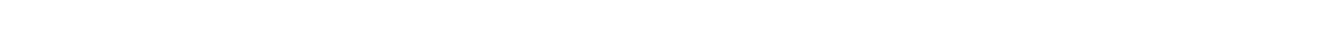# 2.3.5 Application of Conservation Laws

### Application of Conservation Laws

• All particle interactions must obey a set of conservation laws. These are conservation of:
• Charge, Q
• Baryon number, B
• Lepton Number, L
• Strangeness, S
• Energy (or mass-energy)
• Momentum
• However, strangeness does not need to be conserved in weak interactions. It can change by either 0, +1 or –1
• Quantum numbers such as Q, B, L and S can only take discrete values (ie. 0, +1, –1, 1/2)
• To know whether a particle interaction can occur, check whether each quantum number is equal on both sides of the equation
• If even one of them, apart from strangeness in weak interactions, is not conserved then the interaction cannot occurExample of a working out what is conserved in Kaon decay. This decay must be through the weak interaction since S is not conserved

#### Worked Example

The lambda nought particle Λ0 is made up from the quarks uds.

Show, in terms of the conservation of charge, strangeness, baryon number and lepton number whether the following interaction is permittedStep 1: Determine conservation of charge

0 = –1 + 1 + 0

Charge is conserved

Step 2: Determine conservation of strangeness

Λ0 has an s quark, so must have a strangeness of –1

–1 = 0 + 0 + 0

Strangeness is not conserved

Step 3: Determine conservation of baryon number

Λ0 is a baryon since it has 3 quarks, so must have a baryon number of +1

+1 = 0 + 0 + 0

Baryon number is not conserved

Step 4: Determine conservation of lepton number

Λ0 is a baryon, so must have a lepton number of 0

0 = 0 + (–1) + 1

Lepton number is conserved

Step 5: Conclusion

Since the baryon number is not conserved, this interaction is not permitted

#### Worked Example

The equation for β decay isUsing the quark model of beta decay, prove that the charge is conserved in this equation.

#### Exam Tip

Note:

• Quantum numbers for any exotic particles will be given in the question
• All the rest can be found on the datasheet or can be deduced using the information provided### Author: Ashika

Ashika graduated with a first-class Physics degree from Manchester University and, having worked as a software engineer, focused on Physics education, creating engaging content to help students across all levels. Now an experienced GCSE and A Level Physics and Maths tutor, Ashika helps to grow and improve our Physics resources.
CloseClose

### Download all our Revision Notes as PDFs

Try a Free Sample of our revision notes as a printable PDF.

Already a member?